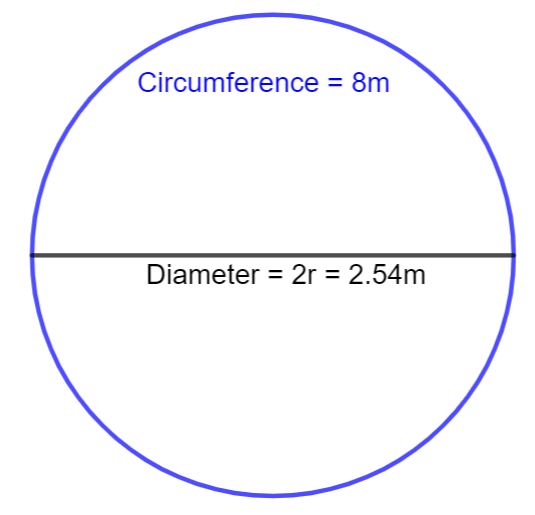Courses
Courses for Kids
Free study material
Offline Centres
MoreLast updated date: 04th Dec 2023
Total views: 281.4k
Views today: 5.81k

# The circumference of the circle is 8m. What is its diameter?Verified
281.4k+ views
Hint: In this problem, we have to find the diameter of a circle whose circumference is given. We know that for a circle with circumference, we can find the diameter. We know that the circumference of a circle is $2\pi r$, we can now equate the given circumference value to the formula of the circumference to find the value of r which is the radius of the circle. We can double the radius which is the diameter of the circle.

We know that the given circumference of the circle is 8 m.
We know that the circumference of the circle is,
Circumference of the circle = $2\pi r$……. (1)
We know that, we are given circumference value is,
$\Rightarrow circumference=8m$ ……….. (2)
We can now equate (1) and (2), we get
$\Rightarrow 2\pi r=8$
We know that the value of $\pi =3.14$, we can simplify the above step, we get
$\Rightarrow r=\dfrac{8}{3.14\times 2}=1.27m$
We can now double the radius, which is the diameter, we get
$\Rightarrow d=2\times r=2\times 1.27=2.54$Therefore, the diameter of a circle with the circumference 8 m is 2.54 m.

Note: We should always remember some formulas in geometry, such as area or circumference of the circle. We should remember that the circumference of the circle is $2\pi r$. We should also know the value of $\pi$ = 3.14 to substitute in these types of problems, whenever required. We should also remember that, we have to mention the unit after finding the answer for it.